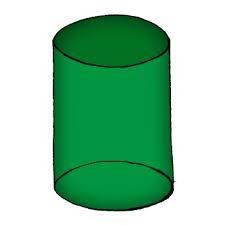# Calculate 5115

In the rotating cylinder it is given: V = 120 cm3, v = 4 cm. Calculate r, S mantle.

r =  3.0902 cm
S2 =  77.665 cm2

### Step-by-step explanation:Did you find an error or inaccuracy? Feel free to write us. Thank you!

Tips to related online calculators
Pythagorean theorem is the base for the right triangle calculator.
Tip: Our volume units converter will help you with the conversion of volume units.

#### You need to know the following knowledge to solve this word math problem:

We encourage you to watch this tutorial video on this math problem: